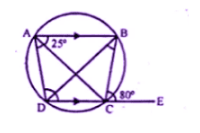Guru

# Ques 3(b) In the figure (if) given below, AB is parallel to DC, ∠BCE = 80° and ∠BAC = 25°. Find: (i) ∠CAD (ii) ∠CBD (iii) ∠ADC

• 0

This is an important and exam oriented question from Chapter name- circles
Topic – Angle properties of circles
Chapter number- 15

This ques has been asked in 2008 question paper

In the figure we have AB is parallel to DC, ∠BCE = 80° and ∠BAC = 25°

Niw we have to Find:
ICSE Avichal publication
Understanding ICSE Mathematics
Question 3(b)

Share

1. (b) in the figure, AB ∥DC

∠BCE = 80o and ∠BAC = 25o

ABCD is a cyclic Quadrilateral and DC is

Production to E(i) Ext, ∠BCE = interior ∠A

∠CAD = 80o – 25o = 55o

(Alternate angels)

∠CBD = 55o

(iii) ∠BAC = ∠BDC

(Angles in the same segments)

∠BDC = 25o

(∠BAC = 25o)

Now AB ∥ DC and BD is the transversal

∠BDC = ∠ABD

∠ABD = 25o

∠ABC = ∠ABD + ∠CBD = 25+ 55o = 80o

But ∠ABC + ∠ADC = 180o

(opposite angles of a cyclic quadrilateral)